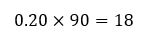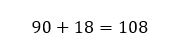# How to add percentages using decimals

Want to find out how to add percentages using decimals? We've provided a step-by-step guide of how to add percentages using decimals to teach you everything you need to know.## Step 1: Change the percentage to decimal by moving two decimal places to the left

For example, if we want to add 15% of 80 to it, we first change 15% to decimal.

## Step 2: Multiply the decimal with the given base value

So, multiply 80 by 0.15.

## Step 3: Add the value from Step 2 to the given base value

Hence, we add 12 and 80.

## Examples of adding percentages using decimals

Q1) What would be the value if we increase 90 by 20%?

Let’s change 20% to decimal by moving two decimal places to the left:This means that 20% is equal to 0.20 in decimal.

Now let’s multiply 0.20 to 90 to find what needs to be added to 90.Once we find 20% of 90, we now add 18 to the base value – 90.So, we have 108 if we increase 90 by 20%.

#### Join today

The fastest way to practice

Unlock our complete testing platform and improve faster that ever.